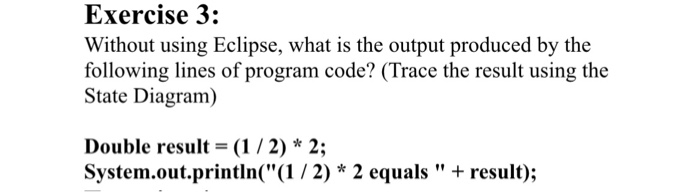# Question & Answer: Without using Eclipse, what is the output produced by the following lines of program code? (Trace the result using the State Diagram) Double result = (1/…..Without using Eclipse, what is the output produced by the following lines of program code? (Trace the result using the State Diagram) Double result = (1/2)* 2: System.out.println(“(1/2)* 2 equals” result);

Answer: I am assuming Double is a primitive data type it means double. If it is a primitive data type in this case then output should be below

Don't use plagiarized sources. Get Your Custom Essay on
Question & Answer: Without using Eclipse, what is the output produced by the following lines of program code? (Trace the result using the State Diagram) Double result = (1/…..
GET AN ESSAY WRITTEN FOR YOU FROM AS LOW AS \$13/PAGE

(1/2) * 2 equals 0.0

Below first (1/2) value calculate. So it will produce 0 instead of 0.5 because both values are integers here.

Case 2

If Double is wrapper class then there is compile error. We should convert the value from int to double.. So in this case code should like below

Double result = (double)(1/2) * 2;

System.out.println(“(1/2) * 2 equals “+result);

In this case also, same output will be printed.

(1/2) * 2 equals 0.0